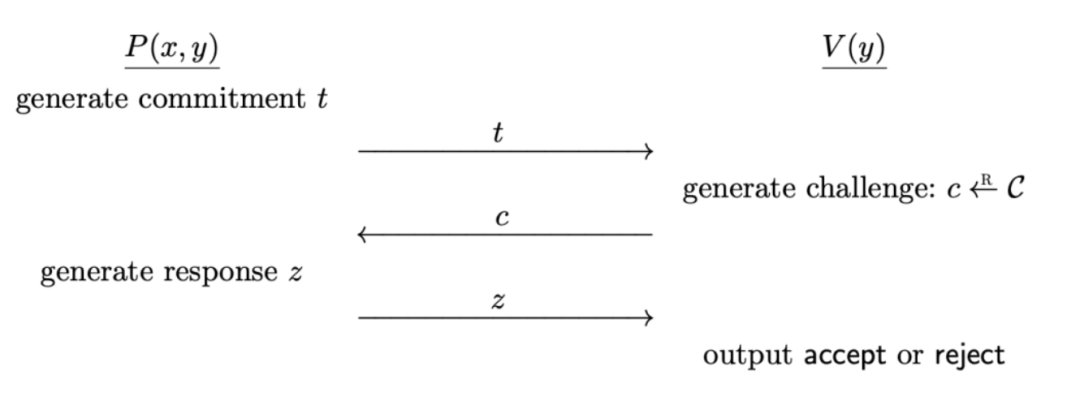## Sigma协议9

P是一个叫证明的交互式协议，其输入为一个witness-statement对$(x,y)\in R$. V是一个叫验证的交互式协议，其输入为一个statement，$y \in R$.

P和V交互过程为：

1. 首先，P计算一个承诺(commitment) t ，将其发送给V；
2. 在收到来自P的消息后，V在有限的挑战空间C中随机选取一个挑战元素(challenge) c，并将其发送给P ；
3. 在接收到来自V的挑战后， P计算出一个反馈(response) z ,将其发送给V
4. 在收到了来自P的反馈后, V输出accept或者reject。### 举例说明

2）P选择随机数$r ∈ z_q$，计算$a = g^r\ mod\ p$, P将a值发送给V

3） V选择随机数challenge e，V将e值发送给P；

4） P计算$z = r + ex\ mod\ q$, 将z值发送给V，

5） V判断 $g^z=? =ah^e\ mod\ p$是否成立，若成立，则V接受认为P确实知道正确的x.

sigma协议又称为诚实验证者的（特殊）零知识证明。即假设验证者是诚实的。这个例子类似Schnorr身份认证协议，只是后者通常采用非交互的方式。

### 零知识性 (special honest verifier zk)

V既不能从协议中知道x的值，而且还不能向第三者，证明V知道这个秘密（即V无法冒充P）。也就是V从协议中什么也没学到（除了P知道x之外）。

## Fiat-Shamir变换

2）P选择随机数$r ∈z_q$ ，计算$a = g^r\ mod\ p$, P将a值发送给V

3）P计算 $e = Hash(h, a)$；

4） P计算$z = r + ex\ mod\ q$, 将z值发送给V，

5） V判断 $g^z=? = ah^e\ mod\ p$是否成立，同时检验e的哈希结果是否正确，都通过后，则V接受认为P确实知道正确的x.

## 小结

### 相关阅读

Schorr签名与椭圆曲线 Schorr签名与椭圆曲线

• 发表于 2021-05-05 12:49
• 阅读 ( 2751 )
• 学分 ( 5 )
• 分类：入门/理论